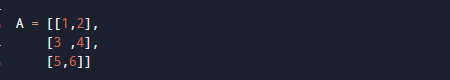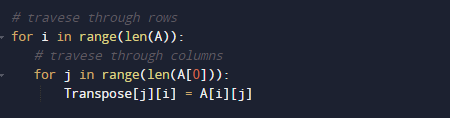# Python Program to Transpose a Matrix

In this tutorial you will learn about the Python Program to Transpose a Matrix and its application with practical example.

In this tutorial, we will learn to create a Python Program to Transpose a Matrix using python Programming.

## Prerequisites

Before starting with this tutorial we assume that you are best aware of the following Python programming topics:

• Python Operator.
• Basic Input and Output
• Basic Python  programming.
• Python Data type.
• Python in-built Functions.
• Loops in Python.
• List in Python.

## Transpose of a Matrix.

The transpose of a matrix is simply a overturn version of Original matrix. We can transpose a matrix by transpose its rows with its columns.

Original MatrixTranspose MatrixIn Mathematics in linear algebra,Transpose of a matrix is actually an operator that flips a matrix above its diagonal by switching the row and column.

## Python Program to Transpose a Matrix.

In this program we create a program that will Transpose a Matrix using nested for loop . We would first declared and initialized the required variables. Next, we would prompt user to input the values  later we will Transpose the matrix.

## Output

Before Transpose.After Transpose.In our program, we have using nested “For” loops for traverse through each row and column. And after traversing at each point we replace the values of ” A[i][j]” to Transpose[j][i].

In the above program, we have first declared and initialized a set variables required in the program.

• A = it will hold Original matrix
• Transpose= it will hold transpose matrix.
• t=it will show Transpose matrix.

Original Matrix.set default value of Transpose to zero.Now , we will Transpose the matrix is by exchanging the rows and columns. Transpose of a matrix is done by changing elements of rows to columns into columns to rows. Or we can say, transpose of T[][] is obtained by changing A[i][j] to A[j][i].Simply If we changes the rows value of a matrix with the value of column of the same matrix, called Transpose of a matrix.

Original Matrix.Transpose Matrix.So here we learnt to transpose a matrix using nested for loop.

In this tutorial we have learn about the Python Program to Transpose a Matrix and its application with practical example. I hope you will like this tutorial.